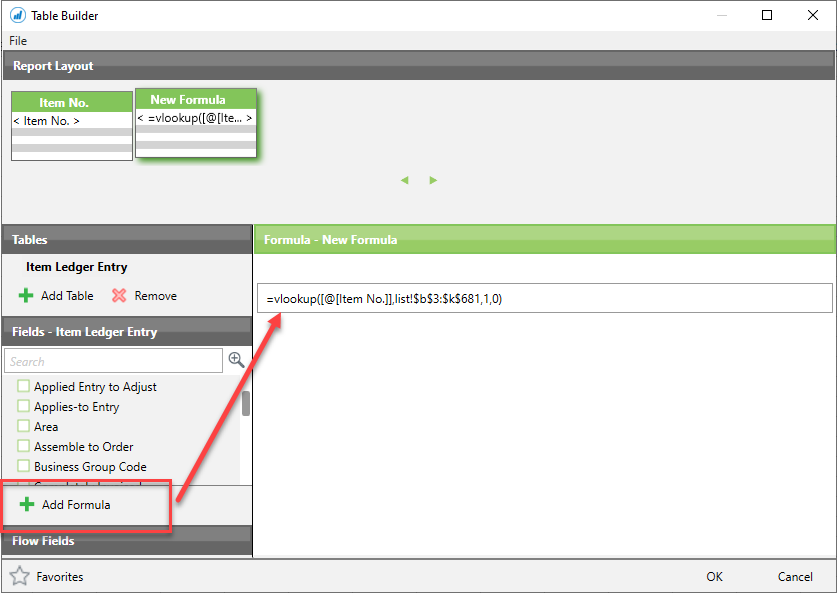Trying to write a vlookup within table builder but it isn't working??

The lookup value is in the Table builder I am creating but the table array is on another worksheet. (called List)

=np("eval","=vlookup([@[Item No.]],list!\$b\$3:\$k\$681,1,0)")

#### 1 comment

•Bryan Robinson

If you are building it directly in the TableBuilder, it will need to use the NP(Formula) function.  If you build the VLOOKUP in the "Add Formula" section of the Table Builder, you just need to put in the VLOOKUP (no NP(Eval))